Genetic Programming Genetic Algorithms 2 Definition Genetic Programming

• Slides: 33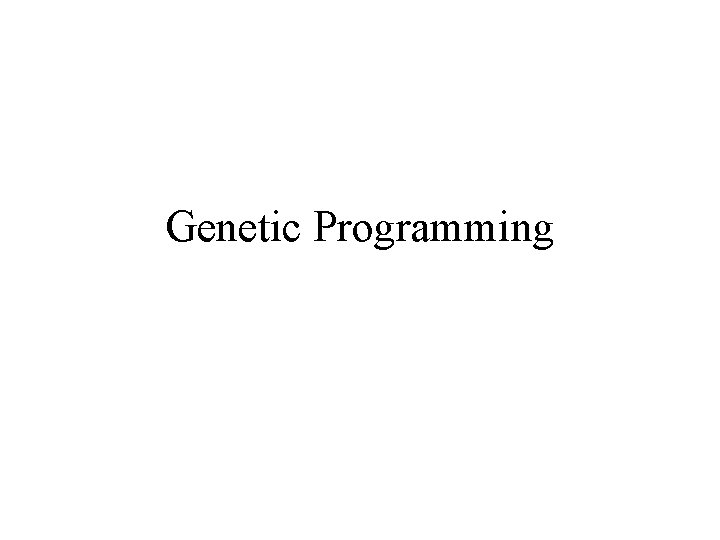Genetic Programming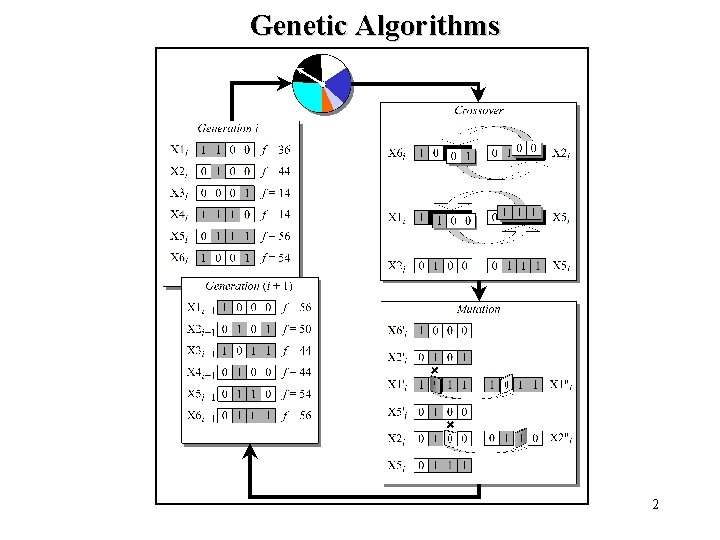Genetic Algorithms 2Definition: Genetic Programming One of the central problems in computer science is how to make computers solve problems without being explicitly programmed to do so. n Genetic programming offers a solution through the evolution of computer programs by methods of natural selection. nn Genetic programming is a recent development in the area of evolutionary computation. It was greatly stimulated in the 1990 s by John Koza. n According to Koza, genetic programming searches the space of possible computer programs for a program that is highly fit for solving the problem at hand.Evolving Programs n Any computer program is a sequence of operations (functions) applied to values (arguments), but different programming languages may include different types of statements and operations, and have different syntactic restrictions.n Since genetic programming manipulates programs by applying genetic operators, a programming language should permit a computer program to be manipulated as data and the newly created data to be executed as a program. For these reasons, LISP was chosen as the main language for genetic programming. n Assembly Language is also used nLISP structure LISP has a highly symbol-oriented structure. Its basic data structures are atoms and lists. An atom is the smallest indivisible element of the LISP syntax. The number 21, the symbol X and the string “This is a string” are examples of LISP atoms. A list is an object composed of atoms and/or other lists. LISP lists are written as an ordered collection of items inside a pair of parentheses.LISP S-expressions For example, the list ( (* A B) C) calls for the application of the subtraction function ( ) to two arguments, namely the list (*A B) and the atom C. First, LISP applies the multiplication function (*) to the atoms A and B. Once the list (*A B) is evaluated, LISP applies the subtraction function ( ) to the two arguments, and thus evaluates the entire list ( (* A B) C).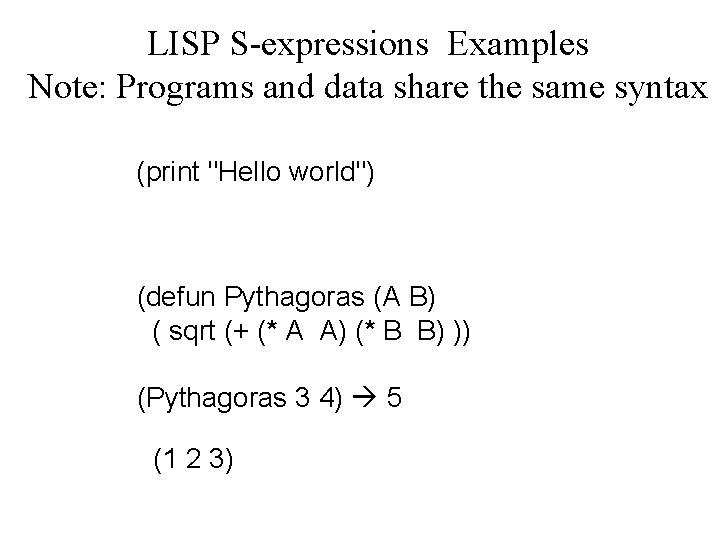LISP S-expressions Examples Note: Programs and data share the same syntax (print "Hello world") (defun Pythagoras (A B) ( sqrt (+ (* A A) (* B B) )) (Pythagoras 3 4) 5 (1 2 3)Graphical representation of LISP S-expressions n Both atoms and lists are called symbolic expressions or S-expressions. In LISP, all data and all programs are S-expressions. This gives LISP the ability to operate on programs as if they were data. In other words, LISP programs can modify themselves or even write other LISP programs. This remarkable property of LISP makes it very attractive for genetic programming.LISP S-expression ( (*A B) C) Any LISP S-expression can be depicted as a rooted point-labelled tree with ordered branches.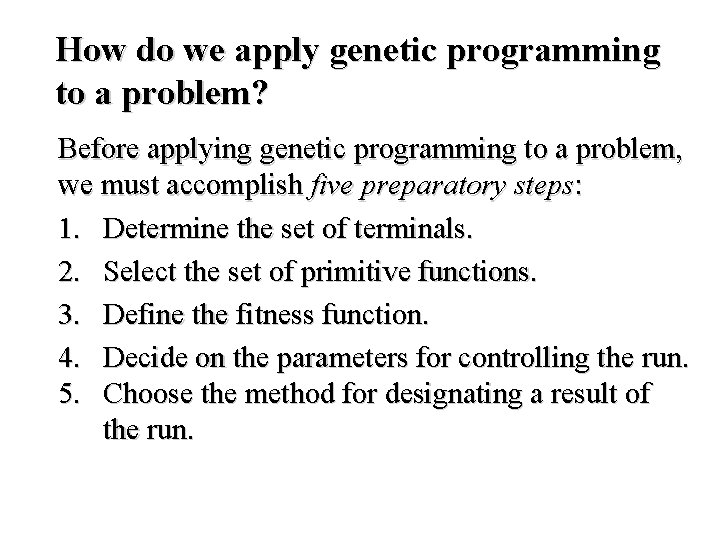How do we apply genetic programming to a problem? Before applying genetic programming to a problem, we must accomplish five preparatory steps: 1. Determine the set of terminals. 2. Select the set of primitive functions. 3. Define the fitness function. 4. Decide on the parameters for controlling the run. 5. Choose the method for designating a result of the run.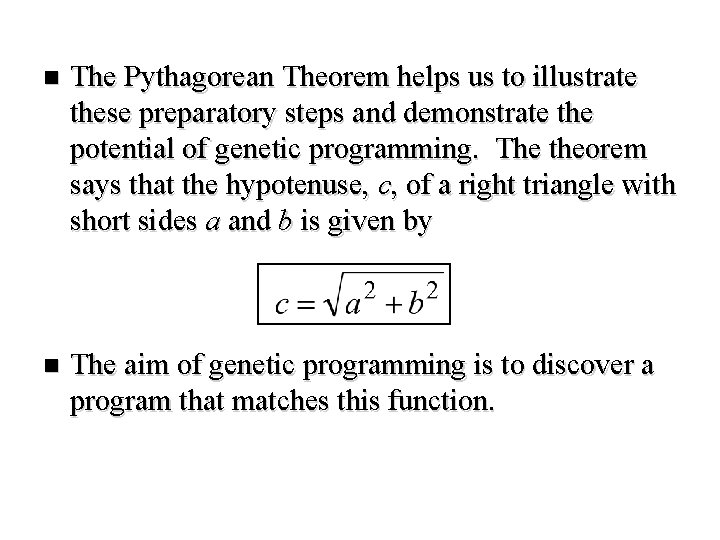n The Pythagorean Theorem helps us to illustrate these preparatory steps and demonstrate the potential of genetic programming. The theorem says that the hypotenuse, c, of a right triangle with short sides a and b is given by n The aim of genetic programming is to discover a program that matches this function.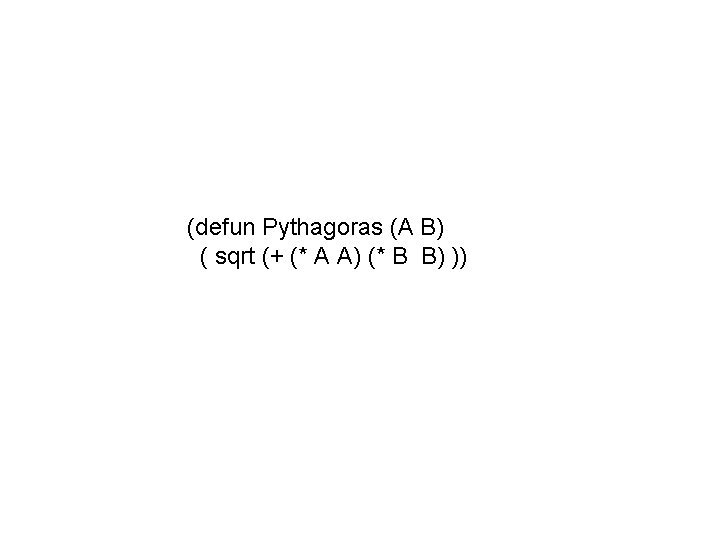(defun Pythagoras (A B) ( sqrt (+ (* A A) (* B B) ))n To measure the performance of the as-yetundiscovered computer program, we will use a number of different fitness cases. The fitness cases for the Pythagorean Theorem are represented by the samples of right triangles in Table. These fitness cases are chosen at random over a range of values of variables a and b.Step 1: Determine the set of terminals. The terminals correspond to the inputs of the computer program to be discovered. Our program takes two inputs, a and b. Step 2: Select the set of primitive functions. The functions can be presented by standard arithmetic operations, standard programming operations, standard mathematical functions, logical functions or domain-specific functions. Our program will use four standard arithmetic operations +, , * and , and one mathematical function sqrt.Step 3: Define the fitness function. A fitness function evaluates how well a particular computer program can solve the problem. For our problem, the fitness of the computer program can be measured by the error between the actual result produced by the program and the correct result given by the fitness case.Step 4: Decide on the parameters for controlling the run. For controlling a run, genetic programming uses the same primary parameters as those used for GAs. They include the population size and the maximum number of generations to be run. Step 5: Choose the method for designating a result of the run. It is common practice in genetic programming to designate the best-so-far generated program as the result of a run.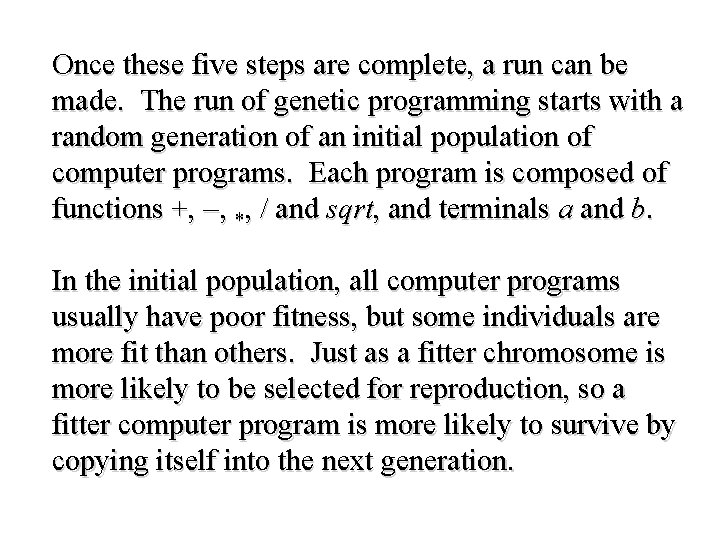Once these five steps are complete, a run can be made. The run of genetic programming starts with a random generation of an initial population of computer programs. Each program is composed of functions +, , *, and sqrt, and terminals a and b. In the initial population, all computer programs usually have poor fitness, but some individuals are more fit than others. Just as a fitter chromosome is more likely to be selected for reproduction, so a fitter computer program is more likely to survive by copying itself into the next generation.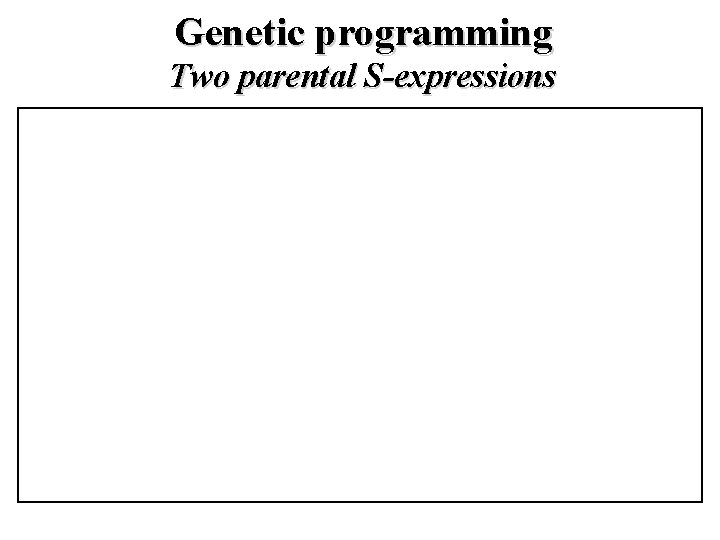Genetic programming Two parental S-expressions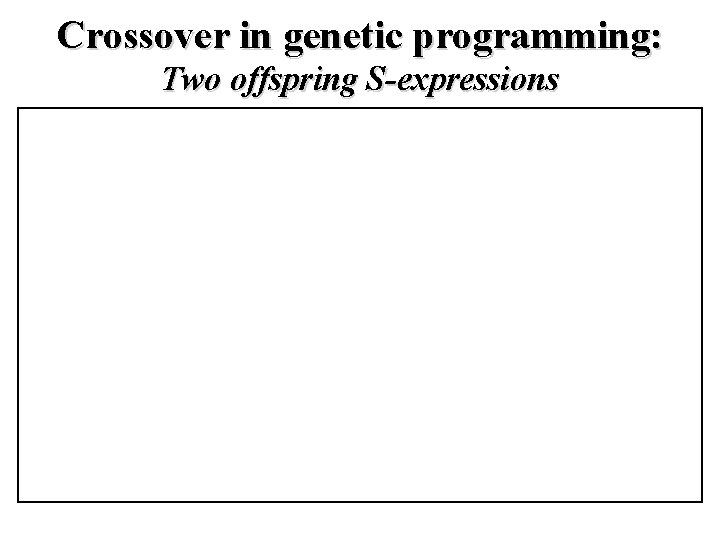Crossover in genetic programming: Two offspring S-expressionsMutation in genetic programming A mutation operator can randomly change any function or any terminal in the LISP S-expression. Under mutation, a function can only be replaced by a function and a terminal can only be replaced by a terminal.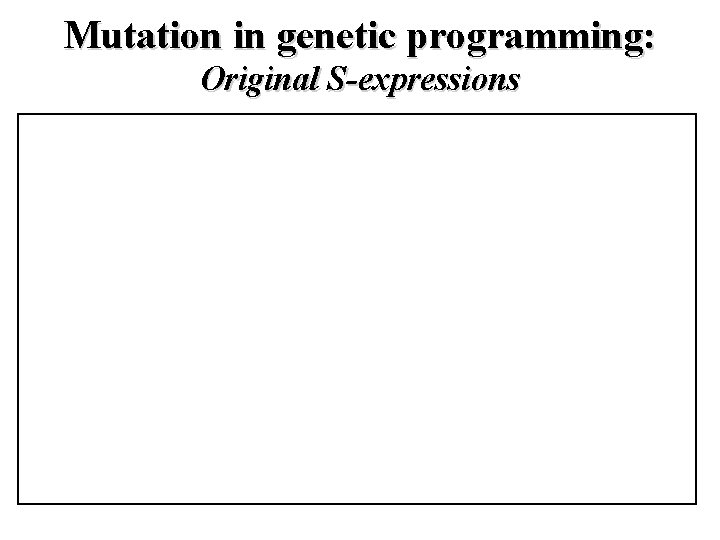Mutation in genetic programming: Original S-expressions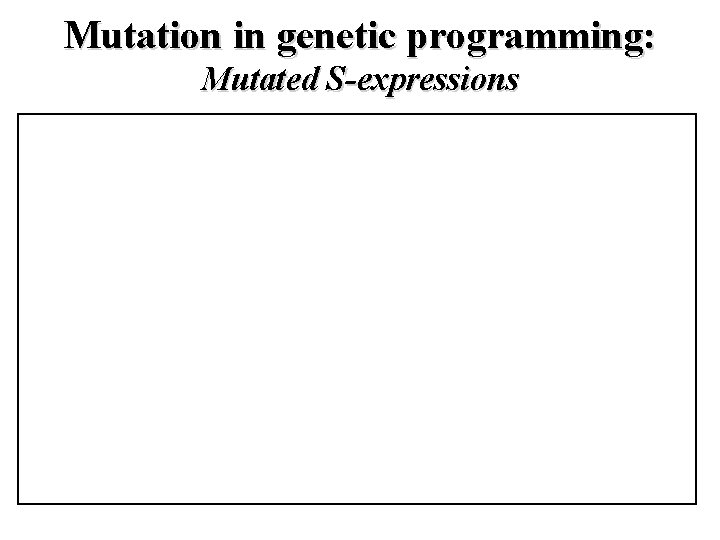Mutation in genetic programming: Mutated S-expressions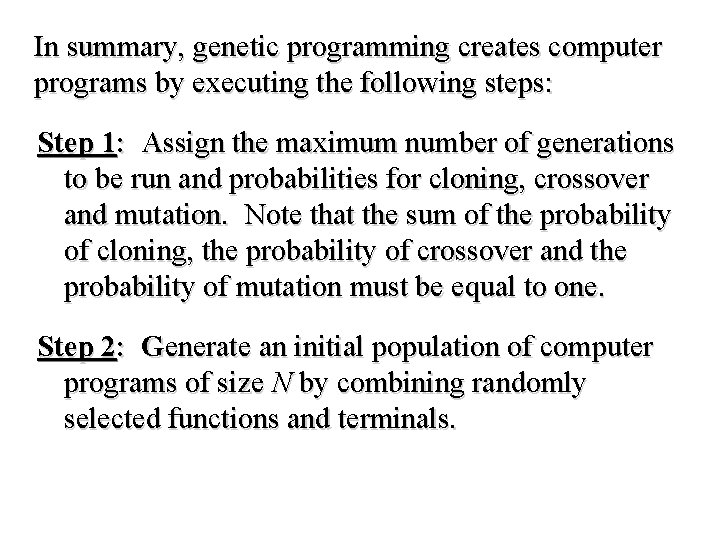In summary, genetic programming creates computer programs by executing the following steps: Step 1: Assign the maximum number of generations to be run and probabilities for cloning, crossover and mutation. Note that the sum of the probability of cloning, the probability of crossover and the probability of mutation must be equal to one. Step 2: Generate an initial population of computer programs of size N by combining randomly selected functions and terminals.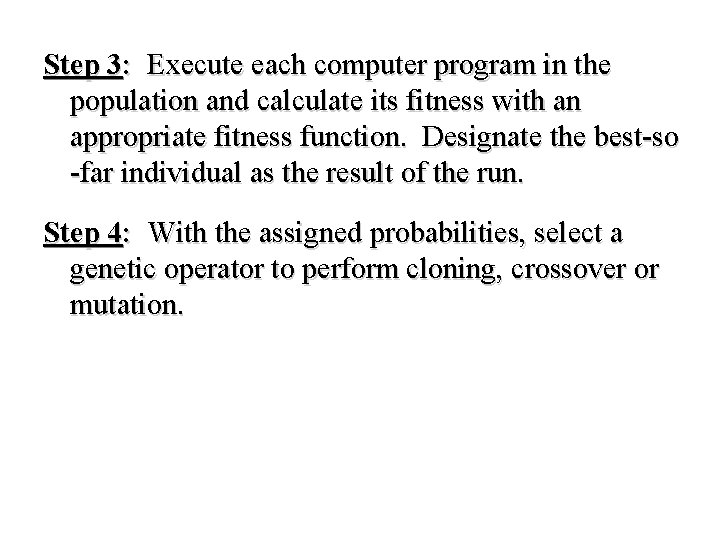Step 3: Execute each computer program in the population and calculate its fitness with an appropriate fitness function. Designate the best-so -far individual as the result of the run. Step 4: With the assigned probabilities, select a genetic operator to perform cloning, crossover or mutation.Step 5: If the cloning operator is chosen, select one computer program from the current population of programs and copy it into a new population. · If the crossover operator is chosen, select a pair of computer programs from the current population, create a pair of offspring programs and place them into the new population. · If the mutation operator is chosen, select one computer program from the current population, perform mutation and place the mutant into the new population.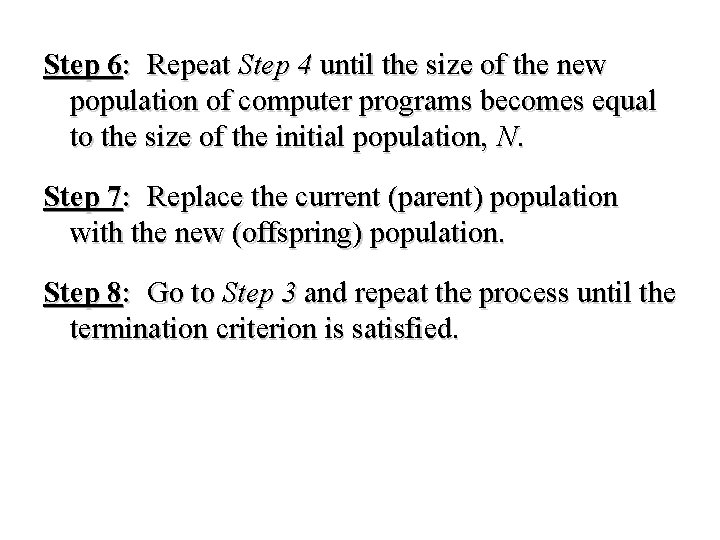Step 6: Repeat Step 4 until the size of the new population of computer programs becomes equal to the size of the initial population, N. Step 7: Replace the current (parent) population with the new (offspring) population. Step 8: Go to Step 3 and repeat the process until the termination criterion is satisfied.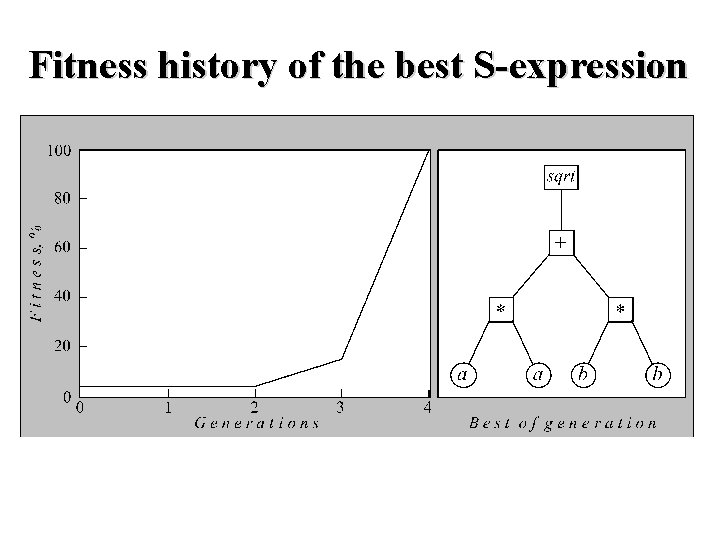Fitness history of the best S-expression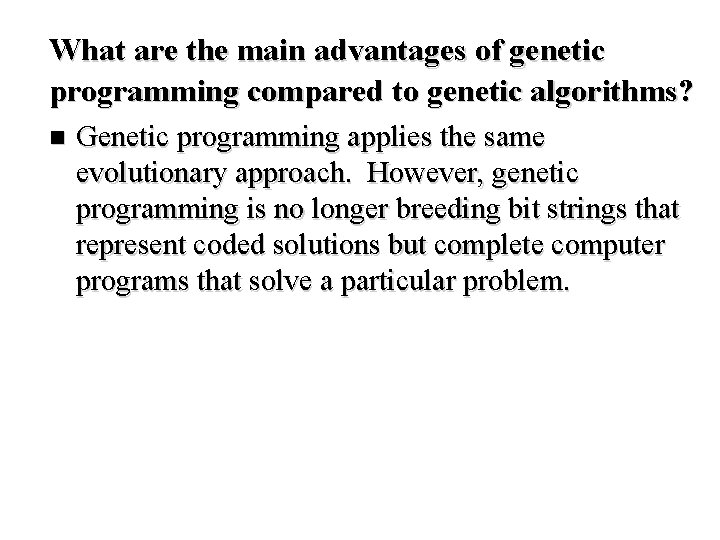What are the main advantages of genetic programming compared to genetic algorithms? n Genetic programming applies the same evolutionary approach. However, genetic programming is no longer breeding bit strings that represent coded solutions but complete computer programs that solve a particular problem.Difficulty n The fundamental difficulty of GAs lies in the problem representation, that is, in the fixed-length coding. A poor representation limits the power of a GA, and even worse, may lead to a false solution.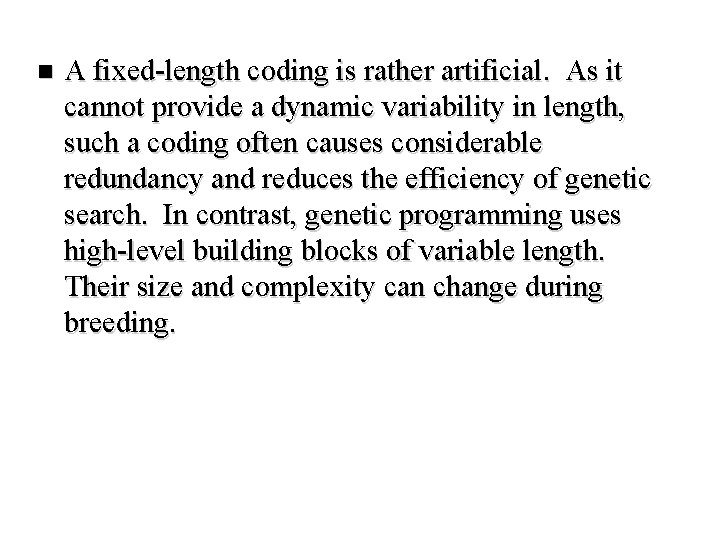n A fixed-length coding is rather artificial. As it cannot provide a dynamic variability in length, such a coding often causes considerable redundancy and reduces the efficiency of genetic search. In contrast, genetic programming uses high-level building blocks of variable length. Their size and complexity can change during breeding.Research n Genetic programming works well in a large number of different cases and has many potential applications.